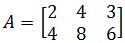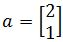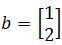# Solution sets of linear systems

### Solution sets of linear systems

#### Lessons

A system of linear equations is homogeneous if we can write the matrix equation in the form $Ax=0$.

A system of linear equations is nonhomogeneous if we can write the matrix equation in the form $Ax=b$.

We can express solution sets of linear systems in parametric vector form.

Here are the types of solutions a homogeneous system can have in parametric vector form:
1. With 1 free variable: $x=tv$
2. With 2 free variables: $x=su+tv$
3. With n free variables: $x=av_1+bv_2+\cdots+nv_n$

Here are the types of solutions a nonhomogeneous system can have in a parametric vector form:
1. With 1 free variable: $x=p+tv$
2. With 2 free variables: $x=p+su+tv$
3. With n free variables: $x=p+av_1+bv_2+\cdots+nv_n$
• Introduction
Solution Set of Linear Systems Overview:
a)
Homogeneous Systems
$Ax=0$
• Trivial, non-Trivial, and general solutions

b)
Solution Sets of Homogeneous Systems
• Parametric Vector Equation with 1 free variable: $x=tv$
• Parametric Vector Equation with 2 free variable $x=su+tv$
• Parametric Vector Equation with more than 2 free variables

c)
NonHomogeneous Systems
$Ax=b$
• General Solutions with an extra vector

d)
Solution Sets of Nonhomogeneous Systems
• Parametric Vector Equation with 1 free variable: : $x=p+tv$
• Parametric Vector Equation with 2 free variables: $x=p+su+tv$
• Parametric Vector Equation with more than 2 free variables

e)
Difference Between Homogeneous and Nonhomogeneous
• Extra $p=$ Translation

• 1.
Solution Sets of Homogeneous Systems
Find the solution set of the homogeneous system in parametric vector form:
$x_1+3x_2+3x_3=0$
$-x_1-3x_2-3x_3=0$
$2x_2+2x_3=0$

• 2.
Find the solution set $Ax=0$ in parametric vector form if:• 3.
Solution Sets of Non-homogeneous Systems
Find the solution set of the nonhomogeneous system in parametric vector form:
$x_1+2x_2-3x_3=2$
$2x_1+x_2-3x_3=4$
$-x_1+x_2=-2$

• 4.
Comparing Homogeneous and Nonhomogeneous Systems
Describe and compare the solution sets of $2x_1+3x_2-5x_3=0$ and $2x_1+3x_2-5x_3=4$

• 5.
Describe and compare the solution sets of $x_1-4x_2+2x_3=0$ and $x_1-4x_2+2x_3=-3$

• 6.
Parametric equation of a line and Translation
Find the parametric equation of the line throughparallel to. Draw all the vectors and the line to show you obtained the line itself geometrically.